Translation of the article prepared in advance of the launch advanced course “Mathematics for Data Science”.

### Introduction

The AdaBoost algorithm can be used to improve the performance of any machine learning algorithm. Machine learning has become a powerful tool for making predictions based on large amounts of data. It has become so popular today that applications with machine learning find application even in everyday tasks. One of the most common examples is product recommendations based on past purchases made by a customer. Machine learning, often called predictive analysis or predictive modeling, can be defined as the ability of computers to learn without explicit programming. Machine learning uses pre-built algorithms to analyze input data to make predictions based on certain criteria.

### What is the AdaBoost algorithm?

In machine learning, boosting is needed to convert weak classifiers to strong ones. A weak learning algorithm or classifier is a learning algorithm that works better than random guessing, and it will work well in case of retraining, because with a large set of weak classifiers, any weak classifier will work better than random sampling. As a weak classifier, the usual `threshold` by a certain sign. If the sign is higher `threshold` (threshold value) than was predicted, it refers to the positive area, otherwise – to the negative.

AdaBoost means “Adaptive Boosting” or adaptive boosting. It turns weak learning algorithms into strong ones to solve classification problems.

The final equation for classification can be as follows:Here fm Is the mth weak classifier, where m responsible for the corresponding weight.

### How does the AdaBoost algorithm work?

AdaBoost can be used to improve algorithm performance machine learning. It works best with weak training algorithms, so such models can achieve accuracy far above random when solving the classification problem. The most common algorithms used with AdaBoost are single-level decision trees. A weak learning algorithm is a classifier or prediction algorithm that works relatively poorly in terms of accuracy. In addition, we can say that weak classifiers are easy to compute, so you can combine many entities of the algorithm to create a stronger classifier using boosting.

If we have a data set in which n is the number of points and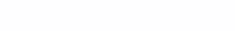Where -1 is the negative class and 1 is the positive. Then the weight of each point will be initialized, as shown below: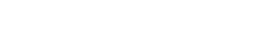Each m in the following expression will vary from 1 to M.
First you need to select a weak classifier with the smallest weighted classification error, applying the classifier to a dataset.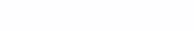Then we calculate the weight of the mth weak classifier, as shown below: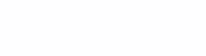Weight is positive for any classifier with an accuracy above 50%. The more weight, the more accurate the classifier. Weight becomes negative when accuracy falls below 50%. Predictions can be combined by inverting the sign. Thus, a classifier with an accuracy of 40% can be converted into a classifier with an accuracy of 60%. So the classifier will contribute to the final prediction, even if it worked worse than random guessing. However, the final result will not change in any way under the influence of a classifier whose accuracy is 50%.

The exponent in the numerator will always be greater than 1 in case of incorrect classification from the classifier with a positive weight. After iteration, the weight of incorrectly classified objects will increase. Classifiers with negative weight will behave in a similar way. There is a difference in sign inversion: the correct classification will become incorrect. The final forecast can be calculated by taking into account the contribution of each classifier and calculating the sum of their weighted forecasts.

The weight for each point will be updated as follows: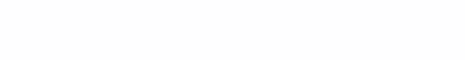Here zm Is a normalizing parameter. It is needed to make sure that the sum of all instance weights is 1.

### Where is the AdaBoost algorithm used?

AdaBoost can be used for face recognition, since it is a standard algorithm for such tasks. It uses a rejection cascade consisting of several layers of classifiers. When the recognition area does not detect faces on any layer, it is rejected. The first classifier in the region discards the negative region to minimize the cost of computing. Although AdaBoost is used to combine weak classifiers, AdaBoost principles are also used to find the best features for each layer in the cascade.

One of the many advantages of the AdaBoost algorithm is that it is easy, fast and easy to program. In addition, it is flexible enough to combine it with any machine learning algorithm without setting parameters, except for parameter T. It is expandable to learning tasks more complicated than binary classification, and is universal enough because it can be used with numerical or textual data.

AdaBoost also has several drawbacks, at least the fact that this algorithm is proved empirically and is very vulnerable to evenly distributed noise. Weak classifiers if they are too weak can lead to poor results and retraining.